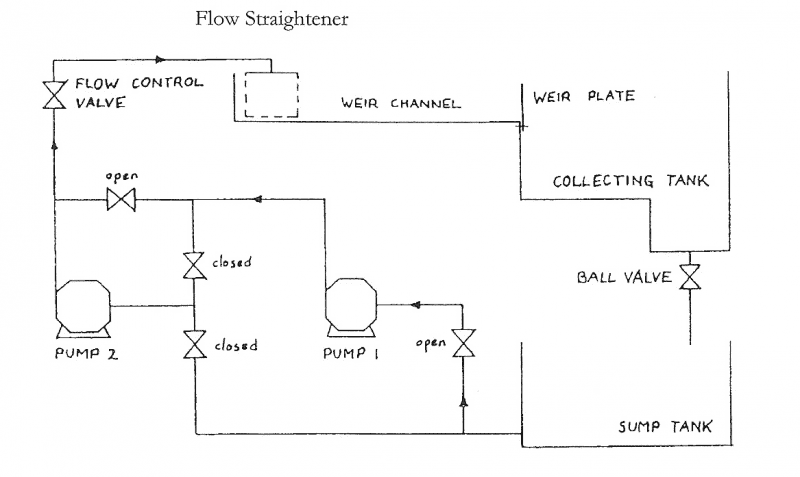# How is the head-flow curve dependant on reynolds number

## Homework Statement

i trying to figure out why the head-flow curve changes at the speeds of 90, 80 and 70 revs and was told that i might want to look into reynolds number for this as the head coefficient is somewhat dependant on this.

Ch=gH/(Nd)^2
Cf=Q/Nd^3
pi=f(Q/Nd^3, re)

## The Attempt at a Solution

Last edited:

Chestermiller
Mentor
Try to put yourself in our place trying to read this post. We have no idea what the geometry is, or where the flow connections are placed. How can we possibly help you, given the information you have provided?

Chet

Try to put yourself in our place trying to read this post. We have no idea what the geometry is, or where the flow connections are placed. How can we possibly help you, given the information you have provided?

Chetthis the diagram given for the experiment layout, the second pump has been isolated for it, a transparent rubber tubing carries the water from the pump through the control valve. This valve controls how much water is let through at a time to the weir channel. Just as the water is about to leave the weir channel it comes into contact with a weir plate where the water gets impeded and needs to push up to get through the triangular gap. Once it gets past the weir plate it enters the collecting tank where that water is collected until it isn’t needed anymore than the ball valve is turned to allow it to enter the sump tank. Finally the water is taken from the sump tank when the pump starts taking in water again.

when the Head-flow was plotted onto a graph for the 3 values of speed, the graph came out with the lines for 70 and 80 revs nearly identical and the 90 revs being down by roughly 0.06 on the head coefficient axis with same gradient as the other 2 lines.

the pump model is a stuart turner type 12 centrifugal pump.
the impeller diameter 0.056m
the water density=1000kg/m3
the water viscosity=1.13x10-3 Kg/ms

Last edited:
Chestermiller
Mentor
You are measuring a centrifugal pump characteristic, and making graphs of the head vs flow rate at different rotational speeds, correct? You are trying to develop a dimensionless plot. Ch is the dimensionless gravitational number, Cf is the dimensionless flow rate, and pi is the dimensionless pressure (how is it defined)? What is the definition of the head coefficient? Can you provide the graphs you are talking about?

Chet

Chestermiller
Mentor
the graph it attached below
as for the head coefficient in this case would be the dimensionless gravitational constant you mentioned. as the equation i had used to calculate the head coefficient was Ch=gH/(Nd)^2
OK. I've looked over your graph, and can see what you mean. First of all, do you think that the difference is that big a deal? If so, do you need to develop a parametric equation to fit all the data, or can you just show the results as Ch vs Cf with Re as a parameter? If it's just the latter, then all you need to do is change the labels on the curves from 70, 80, and 90 to the corresponding ##Re = \frac{\rho ND^2}{\mu}##.

Chet

i see, i dont really think its that big a deal i was just asked to explain find whether Ch was affected by the speed of the impeller and the one who asked me to do so (lecturer) suggested that i look into reynolds number if it did appear to have some change.
thanks for the help.